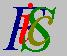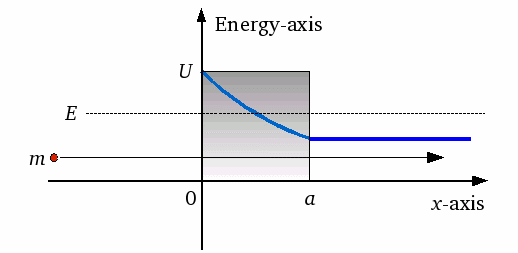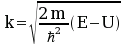Mauro Murzi's pages on Philosophy of Science - Quantum mechanics
Transmission coefficientTunnel effect Alpha radioactivity# [4. Applications]

## Transmission coefficient.A particle moving along the x-axis, with mass m and energy E, approaches from the left a potential barrier with energy U > E. According to quantum mechanics, the probability that the particle penetrates the potential barrier (blue line in the draw) is not null. The transmission coefficient T is the probability that the particle reaches the point a , thus passing the potential barrier and penetrating into the region on the right.

Let φ (x) = e-x k a solution of the first Schrödinger equation applied to the particle inside the potential barrier, where. A rough approximation for the transmission coefficient is given by the quotient of φ (a) by φ (0). This quotient is  e-a k ; the square of its module gives the probability that the particle passes the potential barrier. This probability is, by definition, the transmission coefficient. Thus  T = | φ (a) / φ (0) | 2 = | e - a k |2 = e - 2 a k .Tunnel effect Alpha radioactivity